﻿ 基于RANS/LES混合方法的分离流动模拟Download PDF文章快速检索 高级检索

1. 中国空气动力研究与发展中心 空气动力学国家重点实验室, 绵阳 621000;
2. 中国空气动力研究与发展中心, 绵阳 621000

Simulation of separated flow based on RANS/LES hybrid method
CHEN Hao1,2, YUAN Xianxu1,2, BI Lin1,2, HUA Ruhao1,2, SI Fangfang1,2, TANG Zhigong2
1. State Key Laboratory of Aerodynamics, China Aerodynamics Research and Development Center, Mianyang 621000, China;
2. China Aerodynamics Research and Development Center, Mianyang 621000, China
Abstract: Aircraft maneuvering at high angle of attack and fast pitch leads to large-scale and unsteady vortices in the flow field. Since the traditional Reynolds-Averaged Navier-Stokes (RANS) model cannot accurately simulate the flow field structure, the hybrid RANS/LES model, based on the development trend of international research, is required to accurately simulate the complex separated flow. In this paper, the dual RANS/ Large Eddy Simulatio (LES) hybrid computational model based on zonal mixing and turbulence scale mixing is further developed and applied. Through large static and dynamic turbulent separation flow of typical simplified models, DES-based methods are tested and validated. The correctness and validity of Improved Delayed Detached-Eddy Simulation (IDDES) model in the application to dynamic problems are emphatically studied. The reliability, robustness and accuracy of the numerical simulation method and corresponding calculation software are also tested and verified. Typical examples include supersonic cylindrical bottom flow, transonic square cavity flow and deep stall separation eddy simulation of NACA0015 airfoil. The requirements of three flow types for turbulence simulation methods are provided: steady separated flow, static unsteady separated flow, and dynamic unsteady separated flow. The results show that for static separated flows, DES, DDES and IDDES models are equivalent for static unsteady separated flows, while IDDES models are required for dynamic unsteady separated flows. Meanwhile, the IDDES method developed in this paper can effectively solve the problem of log layer mismatch.
Keywords: unsteady flow    RANS/LES hybrid model    separated flow    detached eddy simulation    IDDES model

Shur和Spalart提出了IDDES(Improved Delayed Detached-Eddy Simulation)方法，不同于通常的LES和(D)DES，IDDES采用了一个依靠壁面距离的新的子网格长度尺度的定义。IDDES方法不仅成功地解决了LLM (Logarithmic-Layer Mismatch)问题，而且在一些包含壁面的复杂流动问题，如后台阶流动，能得到比DDES更好的结果。

1 数值求解方法

1.1 DES类方法

DES方法的基本思想是用统一的湍流模型，以网格尺度和模型中的特征尺度隐式地划分RANS和LES区域。基于SA模型的DES方法是将SA模型中的d转换为网格长度尺度：

 ${l_{{\rm{DES}}}} = {\rm{min}}(d,{C_{{\rm{DES}}}}\varDelta ),\varDelta = {\rm{max}}\{ {\varDelta _x},{\varDelta _y},{\varDelta _z}\}$ （1）

DDES方法是由Spalart和Deck发展，其基本思想是使得计算区域的划分不仅与网格相关，且与涡黏性流场相关。基于SA的DDES的长度尺度定义为

 ${l_{{\rm{DDES}}}} = d - {f_{\rm{d}}}{\rm{max}}(0,d - {C_{{\rm{DES}}}}\varDelta )$ （2）

IDDES方法的提出是为了避免出现对数层不匹配问题，并节省计算量。该方法结合了DDES和壁面模型大涡模拟(Wall-Modeled Large Eddy Simulation, WMLES)方法，在边界层包含湍流脉动时，能够转换为WMLES方法。相对于通常的LES和(D)DES，IDDES采用了新的亚格子尺度的定义，它不仅依靠网格大小，还依靠壁面距离。WMLES模型主要是用于非定常和有湍流的流动中，它通过长度尺度来耦合RANS和LES，有

 ${l_{{\rm{WMLES}}}} = {f_{\rm{B}}}(1 + {f_{\rm{e}}}){l_{{\rm{RANS}}}} + (1 - {f_{\rm{B}}}){l_{{\rm{LES}}}}$ （3）

 ${l_{{\rm{IDDES}}}} = {\tilde f_{\rm{d}}}(1 + {f_{\rm{e}}}){l_{{\rm{RANS}}}} + (1 - {\tilde f_{\rm{d}}}){l_{{\rm{LES}}}}$ （4）

1.2 基于IDDES方法的修正

 $\left\{ {\begin{array}{*{20}{l}} {{\mu _{{\rm{ eff }}}} = {\mu _1} + {\mu _{{\rm{ BLI }}}}}&{\kappa d \le {l_{{\rm{ smg }}}}}\\ {{\mu _{{\rm{ eff }}}} = {\mu _1} + {\mu _{{\rm{ SMG }}}}}&{\kappa d > {l_{{\rm{ smg }}}}} \end{array}} \right.$ （5）

1) 近壁影响修正

 ${f_{{\rm{VD}}}} = 1 - {\rm{exp}}( - {y^ + }/{A^ + })$ （6）

2) 网格拉伸修正

 $\begin{array}{*{20}{l}} {{f_{{\rm{GA}}}} = }\\ {{\rm{cosh}}(\sqrt {\frac{4}{{27}}[{{({\rm{ln}}{a_1})}^2} - {\rm{ln}}{a_1}{\kern 1pt} {\rm{ln}}{a_2} + {{({\rm{ln}}{a_2})}^2}]} )} \end{array}$ （7）

 $\bar \varDelta = \varDelta {f_{{\rm{VD}}}}{\kern 1pt} {f_{{\rm{GA}}}}$ （8）

 ${\mu _{{\rm{SGS}}}} = \bar \rho {({C_{\rm{S}}}\bar \varDelta )^2}|{\tilde S_{ij}}|$ （9）

3) 过渡函数

 ${f_{\rm{d}}} = \frac{1}{2} - \frac{1}{2}{\rm{sin}}\left( {\pi \frac{{{y^ + } - y_{1/2}^ + }}{{d{y^ + }}}} \right)$ （10）

 $\begin{array}{l} {\mu _{{\rm{eff}}}} = \\ \left\{ {\begin{array}{*{20}{l}} {{\mu _1} + {\mu _{{\rm{BLI}}}}}&{{y^ + } < y_{\rm{L}}^ + }\\ {{\mu _l} + {f_{\rm{d}}}{\kern 1pt} {\mu _{{\rm{BLI}}}} + (1 - {f_{\rm{d}}}){\mu _{{\rm{SMG}}}}}&{y_{\rm{L}}^ + < {y^ + } < y_{\rm{R}}^ + }\\ {{\mu _l} + {\mu _{{\rm{SMG}}}}}&{{y^ + } > y_{\rm{R}}^ + } \end{array}} \right. \end{array}$ （11）

2 算例考核 2.1 超声速圆柱底部流动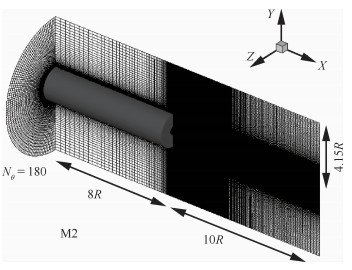图 1 计算网格与计算区域 Fig. 1 Computing mesh and area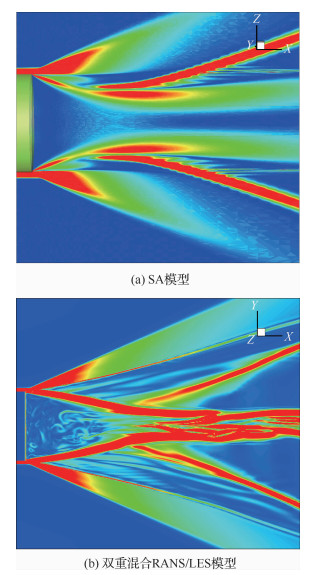图 2 底部瞬时密度梯度 Fig. 2 Instantaneous bottom density gradient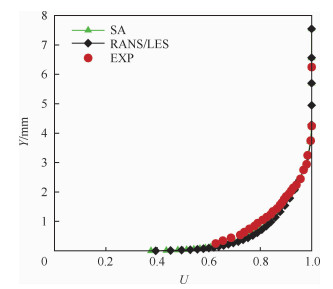图 3 底部前1 mm处平均速度剖面 Fig. 3 Average velocity profile at 1 mm in front of bottom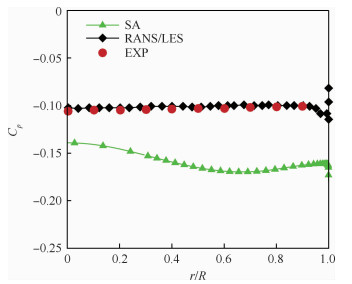图 4 底部平均压力系数分布 Fig. 4 Distribution of average pressure coefficient of bottom图 5 尾流区中心线上平均速度分布 Fig. 5 Average velocity distribution on central line of wake region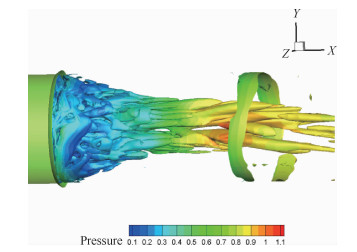图 6 用压力着色的涡量等值面(Q=6，IDDES) Fig. 6 Vorticity equivalent surface colored by pressure (Q=6, IDDES)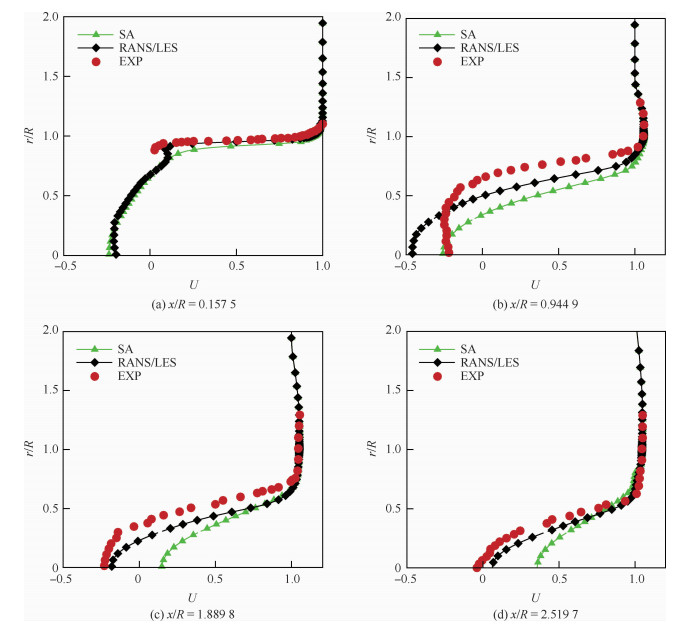图 7 下游不同位置处沿流向速度剖面 Fig. 7 Velocity profiles along flow direction at different downstream locations
2.2 跨声速方腔流动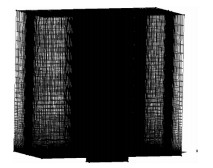图 8 计算网格 Fig. 8 Computing mesh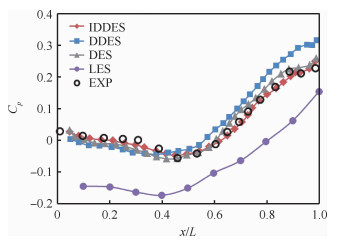图 9 方腔沿流线上的压力系数分布 Fig. 9 Pressure coefficient distribution along square cavity streamline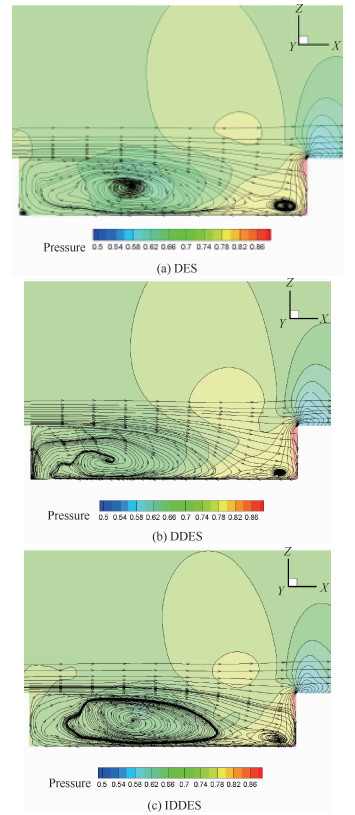图 10 方腔对称截面上的流线 Fig. 10 Streamline on symmetrical section of square cavity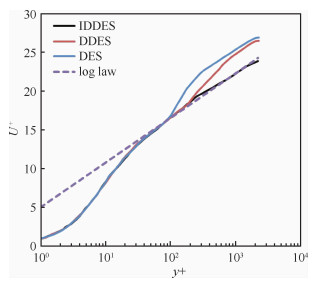图 11 后台阶上壁面平均速度型对比 Fig. 11 Mean velocity profiles comparison on top wall of backward step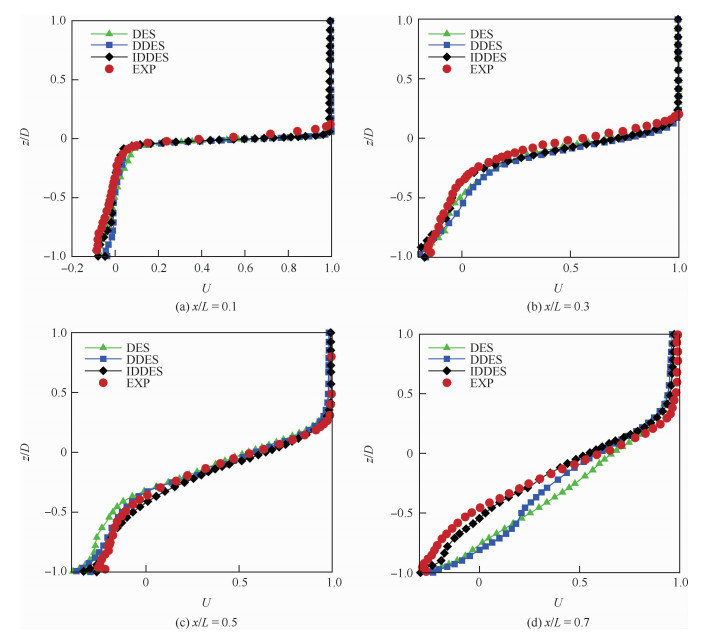图 12 方腔中心对称截面上不同流向位置的流向速度 Fig. 12 Flow velocity of different flow direction positions on central symmetrical section of square cavity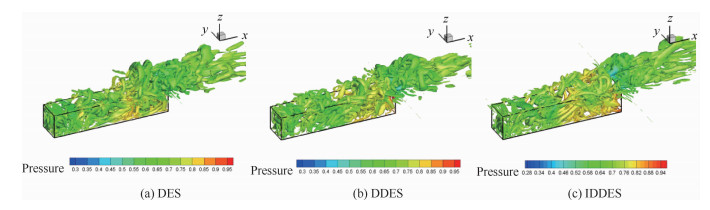图 13 用压力着色的瞬时Q等值面(Q=0.548) Fig. 13 Instantaneous Q isosurface colored by pressure (Q=0.548)
2.3 三维机翼深失速分离涡模拟

 $\alpha (t) = 13.03 + 5.05{\rm{sin}}(\omega {\kern 1pt} {\kern 1pt} t)$ （12）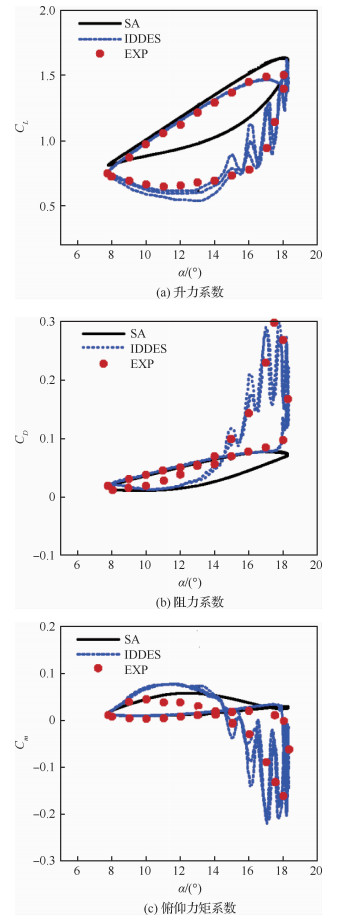图 14 升力、阻力和俯仰力矩系数曲线 Fig. 14 Hysteretic curves of lift, drag and pitching moment coefficients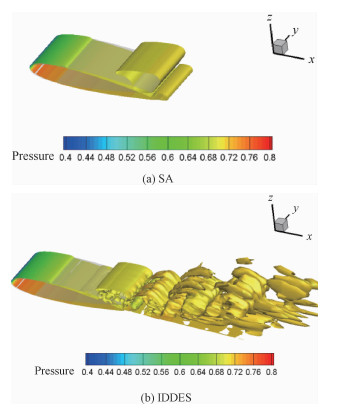图 15 下行迎角16.47°时Q等值面(Q=0.1) Fig. 15 Q equivalent surface at downward angle of attack 16.47° (Q=0.1)
3 结论

1) 基于IDDES方法思想发展的混合模型，通过壁面距离、网格拉伸、过渡函数等修正，与SA方法以及传统的DES和DDES方法相比，经典型算例考核验证，具有对数层匹配性好、复杂分离流动模拟精度高等优势。

2) 对于静态非定常流动，采用基于URANS的DES类模型相对于基于RANS的SA模型有着更好的表现，当流动非定常性不强时，DES、DDES、IDDES 3种模型差别不大；随着流动非定常性增强，IDDES模型的优势逐渐显现。

3) 对于大迎角动态强非定常流动，展向流动现象明显，对NACA0015机翼三维深失速的对比计算表明，SA计算方法对于大迎角动态强非定常流动不再适用，而采用三维IDDES计算方法与试验结果吻合较好。

  张涵信.关于非定常流动的计算问题[C]//第六届全国流体力学会议论文集, 2001. ZHANG H X. On the calculation of unsteady flow[C]//Papers of the Sixth National Conference on Fluid Dynamics, 2001(in Chinese).  张涵信, 周恒.流体力学的基础研究[C]//第六届全国流体力学会议论文集, 2001. ZHANG H X, ZHOU H. Basic research of fluid dynamics[C]//Papers of the Sixth National Conference on Fluid Dynamics, 2001(in Chinese).  周恒. 新世纪对流体力学提出的要求[J]. 自然科学进展, 2000, 10(6): 491-494. ZHOU H. Requirements for hydrodynamics in the new century[J]. Progress in Natural Science, 2000, 10(6): 491-494. (in Chinese) Cited By in Cnki (63) | Click to display the text  SPALART P R, JOU W H. Comments on the feasibility of LES for wings and on the hybrid RANS/LES approach[C]//Advances in DNS/LES, 1st AFOSR International Conference on DNS/LES, 1997.  徐晶磊, 高歌, 杨焱. 基于当地流动结构的RANS/LES混合模型[J]. 航空学报, 2014, 35(11): 2992-2999. XU J L, GAO G, YANG Y. A RANS/LES hybrid model based on local flow structure[J]. Acta Aeronautica et Astronautica Sinica, 2014, 35(11): 2992-2999. (in Chinese) Cited By in Cnki (6) | Click to display the text  MORTON S. High Reynolds number DES simulations of vortex breakdown over a 70 degree delta wing[C]//21st Applied Aerodynamics Conference. Reston: AIAA, 2003.  ALLEN R, MENDONCA F. RANS and DES turbulence model predictions of noise on the M219 cavity at M=0.85[J]. International Journal of Aeroacoustics, 2005, 4: 135-151.  TRAPIER S, DECK S. Delayed detached-eddy simulation and analysis of supersonic inlet buzz[J]. AIAA Journal, 2008, 46(1): 118-131. Click to display the text  FORSYTHE J R, SQUIRES K D. Detached-eddy simulation of the F-15E at high alpha[J]. Journal of Aircraft, 2004, 41(2): 193-200. Click to display the text  GRESCHNER B, THIELE F. Prediction of sound generated by a rod-airfoil configuration using EASM DES and the generalised Lighthill/FW-H analogy[C]//12th AIAA/CEAS Aeroacoustics Conference. Reston: AIAA, 2006.  MENTER F R, KUNTZ M. Adaptation of eddy-viscosity turbulence models to unsteady separated flow behind vehicles[C]//Symposium on the Aerodynamics of Heavy Vehicles: Trucks, Buses and Trains, 2002.  SPALART P R, DECK S. A new version of detached-eddy simulation, resistant to ambiguous grid densities[J]. Theoretical & Computational Fluid Dynamics, 2006, 20(3): 181-195. Click to display the text  SHUR M L, SPALART P R. A hybrid RANS-LES approach with delayed-DES and wall-modelled LES capabilities[J]. International Journal of Heat and Fluid Flow, 2008, 29(6): 1638-1649. Click to display the text  NIKITIN N V, NICOUD F. An approach to wall modeling in large-eddy simulations[J]. Physics of Fluids, 2000, 12(7): 1629. Click to display the text  袁先旭, 邓小兵, 谢昱飞, 等. 超声速湍流流场的RANS/LES混合计算方法研究[J]. 空气动力学学报, 2009, 27(6): 723-728. YUAN X X, DENG X B, XIE Y F, et al. Research on the RANS/LES hybrid method for supersonic-hypersonic turbulence flow[J]. Acta Aerodynamica Sinica, 2009, 27(6): 723-728. (in Chinese) Cited By in Cnki (16) | Click to display the text  KAWAI S, FUJⅡ K. Compact scheme with filtering for large-eddy simulation of transional boundary layer[J]. AIAA Journal, 2008, 46(3): 690-700. Click to display the text  陈琦, 司芳芳, 陈坚强, 等. RANS/LES在超声速突起物绕流中的应用研究[J]. 航空学报, 2013, 34(7): 1531-1537. CHEN Q, SI F F, CHEN J Q, et al. Study of protuberances in supersonic flow with RANS/LES method[J]. Acta Aeronautica et Astronautica Sinica, 2013, 34(7): 1531-1537. (in Chinese) Cited By in Cnki (3) | Click to display the text  HERRIN J L, DUTTON J C. Base bleed experiments with a cylindrical afterbody in supersonic flow[J]. Journal of Spacecraft & Rockets, 1994, 31(6): 1021-1028. Click to display the text  HAASE W, BRAZA M, REVELL A. DESider-A European effort on hybrid RANS-LES modelling[J]. Notes on Numerical Fluid Mechanics & Multidisciplinary Design, 2009, 103: 341-346. Click to display the text  袁先旭.非定常流动数值模拟及飞行器动态特性分析研究[D].绵阳: 中国空气动力研究与发展中心, 2002. YUAN X X. Numerical simulation of unsteady flow and analysis of dynamic characteristics of aircraft[D]. Mianyang: China Aerodynamic Research and Development Center, 2002(in Chinese).  VISBAL M R, SHANG J J S. Numerical investigation of the flow structure around a rapidly pitching airfoil: AIAA-1987-1424[R]. Reston: AIAA, 1987.  RUMSEY C L, ANDERSON W K. Some numerical and physical aspects of unsteady Navier-Stokes computations over airfoils using dynamic meshes: AIAA-1988-0329[R]. Reston: AIAA, 1988.  NIU Y Y, LIOU M S. Numerical simulation of dynamic stall using upwind methods with dual time stepping: AIAA-1997-1000[R]. Reston: AIAA, 1997.  LANDON R H. NACA0012, oscillatory and transient pitching: AGARD-R-702[R]. 2000.  PIZIALI R A. 2-D and 3-D oscillating wing aerodynamics for a range of angles of attack including stall: NASA TM-4632[R]. Washington, D.C.: NASA, 1994.  XIAO Z Z, LIU J. Numerical dissipation effects on massive separation around tandem cylinders[J]. AIAA Journal, 2012, 50(5): 1119-1136. Click to display the text  刘周, 杨云军, 周伟江, 等.使用RANS/LES混合方法模拟翼型大迎角动态非定常分离流动[C]//第十四届全国分离流、旋涡和流动控制会议论文集, 2013. LIU Z, YANG Y J, ZHOU W J, et al. Using RANS/LES hybrid method to simulate dynamic unsteady separated flow of airfoil at high angle of attack[C]//Proceedings of the 14th National Conference on Separated Flow, Vortex and Flow Control, 2013(in Chinese).
http://dx.doi.org/10.7527/S1000-6893.2020.23642

0

#### 文章信息

CHEN Hao, YUAN Xianxu, BI Lin, HUA Ruhao, SI Fangfang, TANG Zhigong

Simulation of separated flow based on RANS/LES hybrid method

Acta Aeronautica et Astronautica Sinica, 2020, 41(8): 123642.
http://dx.doi.org/10.7527/S1000-6893.2020.23642